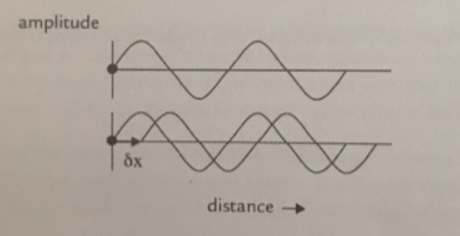# Problem: Two waves shown below with the same amplitude, A, and wavelength, λ, and traveling in the same direction. Initially the sources (dot at the origin) are also at the same point. The source of the second wave is then displaced by a distance δ x. a) For what values of δx will the superposition of the two waves show total constructive interference? Give at least two values in terms of λ. b) For what values of δx will the superposition of the two waves show total destructive interference? Give at least two values in terms of λ.

###### FREE Expert Solution

a)

For constructive interference, the peaks of both waves superimpose, where δx = nλ

99% (107 ratings)###### Problem Details

Two waves shown below with the same amplitude, A, and wavelength, λ, and traveling in the same direction. Initially the sources (dot at the origin) are also at the same point. The source of the second wave is then displaced by a distance δ x.a) For what values of δx will the superposition of the two waves show total constructive interference? Give at least two values in terms of λ.

b) For what values of δx will the superposition of the two waves show total destructive interference? Give at least two values in terms of λ.